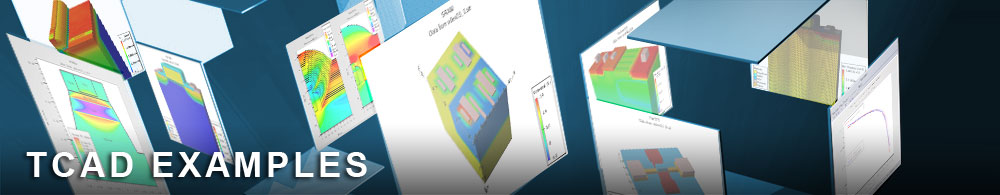Semi-classical versus BQP Quasistatic Capacitance for an N-MOS.

## quantumex06.in : Semi-classical versus BQP Quasistatic Capacitance for an N-MOS.

Requires: S-Pisces/Quantum
Minimum Versions: Atlas 5.28.1.R

This example demonstrates:

• Use of the BQP model.
• Calculation of very low frequency C-V curves using QSCV
• Effects of quantum mechanics on C-V characteristics of NMOS.

In this example we illustrate the effects of quantum mechanics on the quasi-static (i.e. very low frequency) capacitance of an N-MOS capacitor as the gate bias varies, taking the device from being in accumulation through to strong inversion. The main features are the shift in threshold voltage, equality of the capacitances near flat band and in depletion, and the different asymptotic capacitance as the gate voltage becomes large, both +ve and -ve.

The first part invokes the BQP model for both electrons and holes. This is because quantum effects for both electrons and holes are important on the same bias sweep. There is negligible current flow in this device and so CARRIERS=0 mode can be used, which is sufficiently robust that the model can be solved with the gate bias at -3.0 V directly. A structure file is saved at this point as an example of the device in accumulation. By specifying P.Quantum on the output statement the BQP is written to the structure file. The gate bias is then ramped all the way up to 3.0V with QSCV specified on the solve statement in order to calculate and save the quasistatic C-V curves. After the final voltage, another structure file is output as an example of inversion. The quantum effects are only apparent near the Si/SiO2 interface.

The second part does the semi-classical calculation over the same bias range.

Remarks : The BQP calculation is a self-consistent one and can take much longer to run than the equivalent semi-classical one.

To load and run this example, select the Load button in DeckBuild > Examples. This will copy the input file and any support files to your current working directory. Select the Run button in DeckBuild to execute the example.